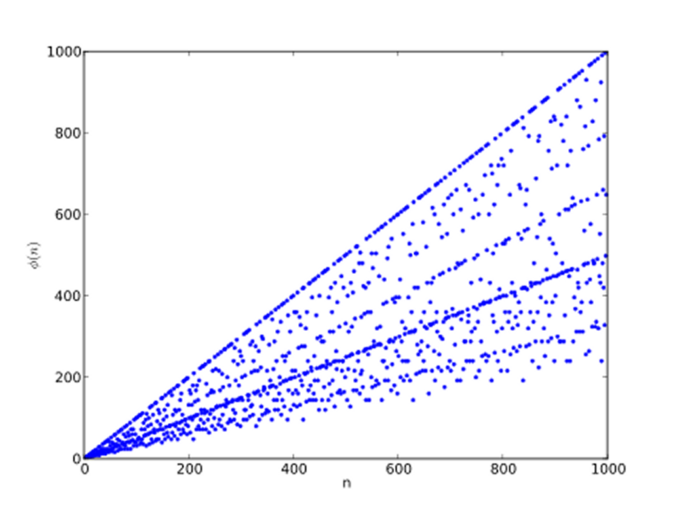# Euler’s Totient Function

Euler’s totient function counts the number of positive integers up to $$n$$ that are relatively prime to $$n$$, where $$1$$ is considered to be relatively prime to all $$n$$.  These numbers are called the totatives of $$n$$.

The totient function is denoted by $$\varphi(n)$$.

$$\varphi(p) = p-1$$, for a prime $$p$$.

Euler’s product formula for the function is :

$$\displaystyle \varphi(n) = n\prod_{p|n}\Bigl(1-\frac 1p \Bigr)$$,

where $$p|n$$ are the distinct primes that divide the given number and $$\prod$$ is the product over them.

For example, $$12 = 2^2 \cdot3$$

$$\displaystyle \varphi(12) = 12\Bigl(1-\frac12 \Bigr)\Bigl(1-\frac 13 \Bigr)$$

$$\displaystyle = 12 \cdot \frac12 \cdot \frac23 = 4$$

The totatives of $$12$$  are $$1, 5, 7$$ and $$11$$.

$$\displaystyle \varphi(13) = 13\Bigl(1-\frac{1}{13}\Bigr)$$

$$\displaystyle = 13 \cdot \frac{12}{13} = 12$$

The totatives of $$13$$ are all the numbers less than $$13$$.

$$\displaystyle \varphi(30) = 30\Bigl(1-\frac12\Bigr)\Bigl(1-\frac13\Bigr)\Bigl(1-\frac15\Bigr)$$

$$\displaystyle = 30 \cdot \frac12 \cdot \frac23 \cdot \frac45 = 8$$

The totatives of $$30$$ are $$1, 7, 11, 13, 17, 19, 23$$ and $$29$$.

The first few values for $$\varphi(n)$$ :

$$\begin{array}{c|cccccccccccc} n&1&2&3&4&5&6&7&8&9&10&11&12 \\ \varphi(n)&1&1&2&2&4&2&6&4&6&4&10&4 \end{array}$$

A graph of the first thousand values :The first thousand values of φ(n). The points on the top line represent φ(p) when p is a prime number, which is p − 1Pietro Battiston (it:User:Toobaz) [CC BY-SA]Euler’s phi function has the important property of being a multiplicative function.  That is, whenever $$m$$ and $$n$$ are two relatively prime integers (gcd$$(m, n) = 1$$) , then

$$\varphi(mn) = \varphi(m)\varphi(n)$$

This aids speed of computation, for example,

$$\varphi(35) = \varphi(5)\varphi(7) = 4\cdot6 = 24$$

Another property of the function is the prime power argument :

$$\varphi(p^\alpha) = p^\alpha-p^{\alpha-1}$$,

for prime $$p$$ and $$\alpha \geq 1$$.

For example,

$$\varphi(2^6) = 2^6-2^5 = 64-32 = 32$$

and

$$\varphi(5^3) = 5^3-5^2 = 125-25 = 100$$.

Gauss established another identity that relates the divisors of $$n$$ to $$n$$ via the formula

$$\displaystyle \sum_{d|n} \varphi(d) = n$$

where $$d|n$$ are the divisors of n and $$\sum \varphi(d)$$ is the sum of the totients of the divisors.

For example, the divisors of $$12$$ are $$1, 2, 3, 4, 6$$ and $$12$$ which have totients $$1, 1, 2, 2, 2, 4$$.

Now, $$1 + 1 + 2 + 2 + 2 + 4 = 12$$ so

$$\displaystyle \sum_{d|12} \varphi(d) = 12$$

For a prime number the sum is very simple.  For example, the divisors of $$13$$ are $$1$$ and $$13$$ which have totients $$1$$ and $$12$$ and $$1 + 12 = 13$$.

Euler generalised Fermat’s Little Theorem.

Euler’s Theorem states :

$$a^{\varphi(n)} \equiv 1\pmod n$$

where $$a$$ and $$n$$ are relatively prime $$(gcd(a, n) = 1)$$.

The special case where $$n$$ is prime is Fermat’s Little Theorem :

$$a^{p-1} \equiv 1 \pmod p$$

Fermat’s Little Theorem

© OldTrout $$2018$$